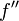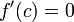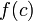# Second derivative test

## Statement

### What this test is for

This test is a partial test (i.e., it may be inconclusive) for determining whether a given critical point for a function is a point of local minimum, point of local maximum, or neither.

### What the test states

Suppose$f$ is a function and$c$ is a point in the interior of the domain of$f$, i.e.,$f$ is defined on some open interval containing$c$. Suppose, further, that$f''$, i.e., the second derivative of$f$, exists at$c$. Suppose also that$f'(c)=0$, so$c$ is a critical point for$f$. Then:

Hypothesis Conclusion$f''(c) < 0$$f$ attains a local maximum value at$c$ (the value is$f(c)$)$f''(c) > 0$$f$ attains a local minimum value at$c$ (the value is$f(c)$)$f''(c) = 0$ The test is inconclusive.$f$ may attain a local maximum value, a local minimum value, have a point of inflection, or have some different behavior at the point$c$.